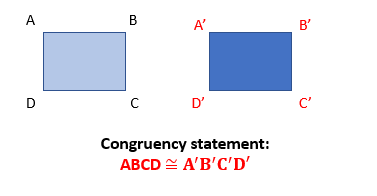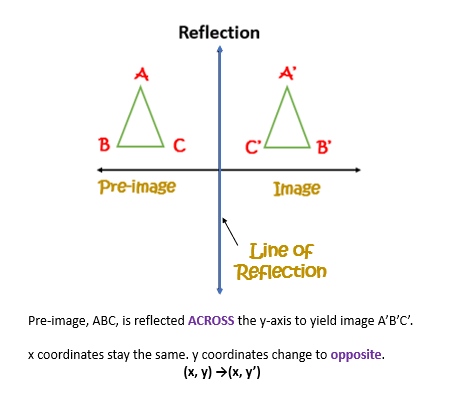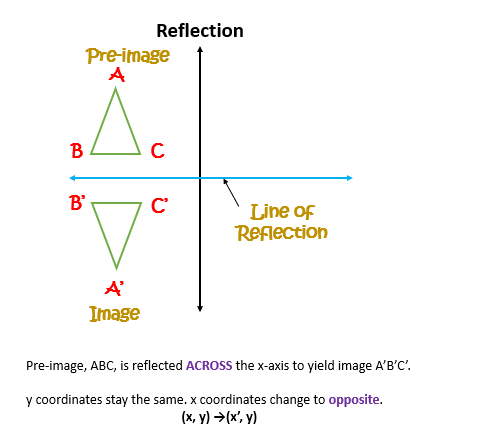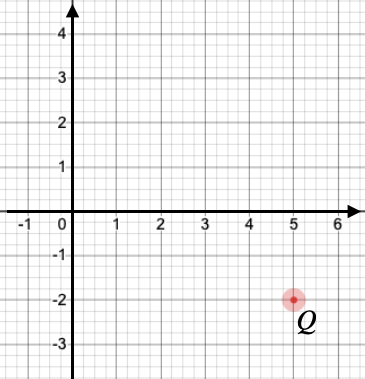## Introduction

• To transform a figure (shape) means changing the size, location, and direction it faces.
• The figure before the transformation is called the pre-image
• The figure after the transformation is called the image
• If the pre-image is labeled as A, then the image would be labeled as A' (pronounced as A prime).
• There are four different types of transformation.
1. Dilation
2. Reflection
3. Rotation
4. Translation

## Congruency

• Two figures are congruent if they are of the same shape and size.
• The image after rotation is congruent with the pre-image.
• The symbol for congruency is ≅## Reflection

• A type of transformation that flips a figure across a line.
• It creates a mirror image (congruent figure). Congruent means the pre-image and the image have the same size and shape.## Solved Examples

Question 1: What is the reflection of a point (2, -7) across the x-axis?

Solution: The reflection of a point (x, y) across the x-axis is the point (x, -y). So, the reflection of the point (2, -7) will be (2, 7).

Question 2: What is the reflection of a point (-3, 12) across the y-axis?

Solution: The reflection of a point (x, y) across the y-axis is the point (-x, y). So, the reflection of the point (-3, 12) will be (3, 12).

Question 3: Find the reflection of a point (-5, 1) across the line $y=x$?

Solution: The reflection of a point (x, y) across the line $y=x$ is the point (y, x). So, the reflection of the point (-5, 1) across $y=x$ will be (1, -5).

Question 4: Point  is obtained after reflection of the point  about the x-axis as shown in the graph. Find the coordinates of the point $P$.Solution: If point $Q\left(x,y\right)$ is reflected over the x-axis, the new point coordinate is ${Q}^{\text{'}}\left(x,-y\right)$. Point  when reflected over the x-axis will produce $Q\left(5,-2\right)$.

## Cheat Sheet

• A reflection flips a figure over a line of reflection to create a mirror image
• Each reflected point of the figure should have the same distance from the line of reflection on the opposite side.
• The reflected image and pre-image are congruent.
• In a coordinate plane, the image can be reflected around both axes.

## Blunder Areas

• Differentiate between pre-image (A) and image (A').
• Differentiate between the x-axis and y-axis as the line of reflection.